# Vector math¶

## Introdução¶

Este tutorial é uma introdução curta e prática à Álgebra Linear e como ela se aplica ao desenvolvimento de jogos. A Álgebra Linear é o estudo de vetores e seus usos. Vetores possuem diversas aplicações em desenvolvimento 2D e 3D, e Godot as usa extensivamente. Desenvolver um bom entendimento de matemática vetorial é essencial para se tornar um bom desenvolvedor de jogos.

Nota

This tutorial is not a formal textbook on linear algebra. We will only be looking at how it is applied to game development. For a broader look at the mathematics, see https://www.khanacademy.org/math/linear-algebra

## Coordinate systems (2D)¶

In 2D space, coordinates are defined using a horizontal axis (x) and a vertical axis (y). A particular position in 2D space is written as a pair of values such as (4, 3).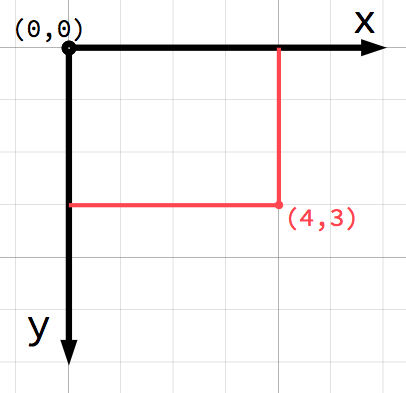Nota

If you’re new to computer graphics, it might seem odd that the positive y axis points downwards instead of upwards, as you probably learned in math class. However, this is common in most computer graphics applications.

Any position in the 2D plane can be identified by a pair of numbers in this way. However, we can also think of the position (4, 3) as an offset from the (0, 0) point, or origin. Draw an arrow pointing from the origin to the point: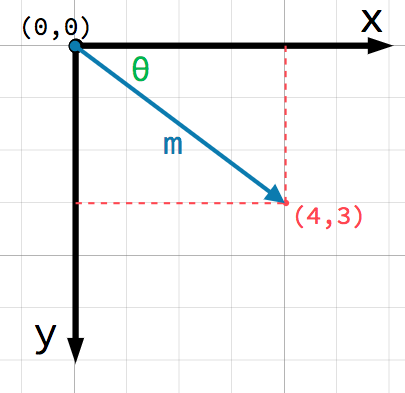This is a vector. A vector represents a lot of useful information. As well as telling us that the point is at (4, 3), we can also think of it as an angle θ and a length (or magnitude) m. In this case, the arrow is a position vector - it denotes a position in space, relative to the origin.

A very important point to consider about vectors is that they only represent relative direction and magnitude. There is no concept of a vector’s position. The following two vectors are identical: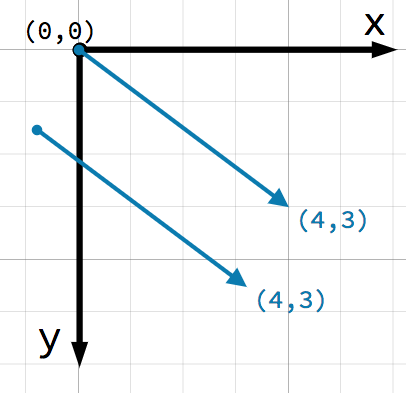Both vectors represent a point 4 units to the right and 3 units below some starting point. It does not matter where on the plane you draw the vector, it always represents a relative direction and magnitude.

## Vector operations¶

You can use either method (x and y coordinates or angle and magnitude) to refer to a vector, but for convenience, programmers typically use the coordinate notation. For example, in Godot, the origin is the top-left corner of the screen, so to place a 2D node named Node2D 400 pixels to the right and 300 pixels down, use the following code:

\$Node2D.position = Vector2(400, 300)

var node2D = (Node2D) GetNode("Node2D");
node2D.Position = new Vector2(400, 300);


Godot supports both Vector2 and Vector3 for 2D and 3D usage, respectively. The same mathematical rules discussed in this article apply to both types.

• Member access

The individual components of the vector can be accessed directly by name.

# create a vector with coordinates (2, 5)
var a = Vector2(2, 5)
# create a vector and assign x and y manually
var b = Vector2()
b.x = 3
b.y = 1

// create a vector with coordinates (2, 5)
var a = new Vector2(2, 5);
// create a vector and assign x and y manually
var b = new Vector2();
b.x = 3;
b.y = 1;


var c = a + b  # (2, 5) + (3, 1) = (5, 6)

var c = a + b;  // (2, 5) + (3, 1) = (5, 6)


We can also see this visually by adding the second vector at the end of the first: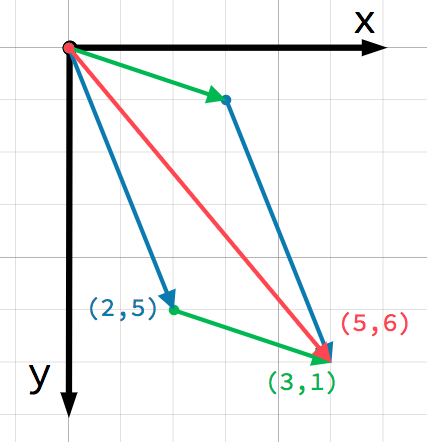Note that adding a + b gives the same result as b + a.

• Scalar multiplication

Nota

Vectors represent both direction and magnitude. A value representing only magnitude is called a scalar.

A vector can be multiplied by a scalar:

var c = a * 2  # (2, 5) * 2 = (4, 10)
var d = b / 3  # (3, 6) / 3 = (1, 2)

var c = a * 2;  // (2, 5) * 2 = (4, 10)
var d = b / 3;  // (3, 6) / 3 = (1, 2)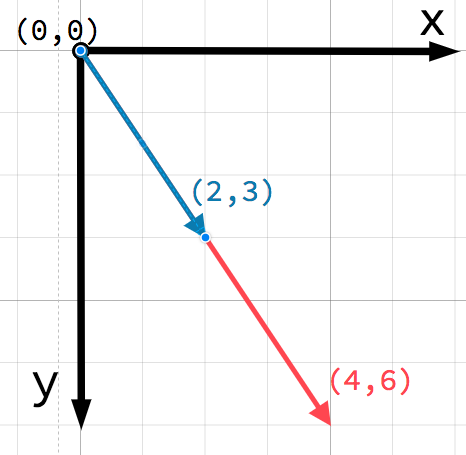Nota

Multiplying a vector by a scalar does not change its direction, only its magnitude. This is how you scale a vector.

## Practical applications¶

Let’s look at two common uses for vector addition and subtraction.

• Movement

A vector can represent any quantity with a magnitude and direction. Typical examples are: position, velocity, acceleration, and force. In this image, the spaceship at step 1 has a position vector of (1,3) and a velocity vector of (2,1). The velocity vector represents how far the ship moves each step. We can find the position for step 2 by adding the velocity to the current position.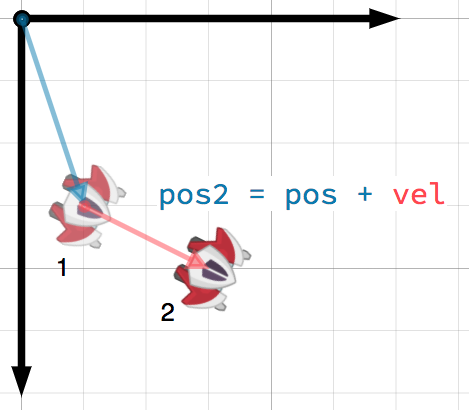Dica

Velocity measures the change in position per unit of time. The new position is found by adding velocity to the previous position.

• Pointing toward a target

In this scenario, you have a tank that wishes to point its turret at a robot. Subtracting the tank’s position from the robot’s position gives the vector pointing from the tank to the robot.Dica

To find a vector pointing from A to B use B - A.

## Unit vectors¶

A vector with magnitude of 1 is called a unit vector. They are also sometimes referred to as direction vectors or normals. Unit vectors are helpful when you need to keep track of a direction.

### Normalization¶

Normalizing a vector means reducing its length to 1 while preserving its direction. This is done by dividing each of its components by its magnitude:

var a = Vector2(2, 4)
var m = sqrt(a.x*a.x + a.y*a.y)  # get magnitude "m" using the Pythagorean theorem
a.x /= m
a.y /= m

var a = new Vector2(2, 4);
var m = Mathf.Sqrt(a.x*a.x + a.y*a.y);  // get magnitude "m" using the Pythagorean theorem
a.x /= m;
a.y /= m;


Because this is such a common operation, Vector2 and Vector3 provide a method for normalizing:

a = a.normalized()

a = a.Normalized();


Aviso

Because normalization involves dividing by the vector’s length, you cannot normalize a vector of length 0. Attempting to do so will result in an error.

### Reflection¶

A common use of unit vectors is to indicate normals. Normal vectors are unit vectors aligned perpendicularly to a surface, defining its direction. They are commonly used for lighting, collisions, and other operations involving surfaces.

For example, imagine we have a moving ball that we want to bounce off a wall or other object: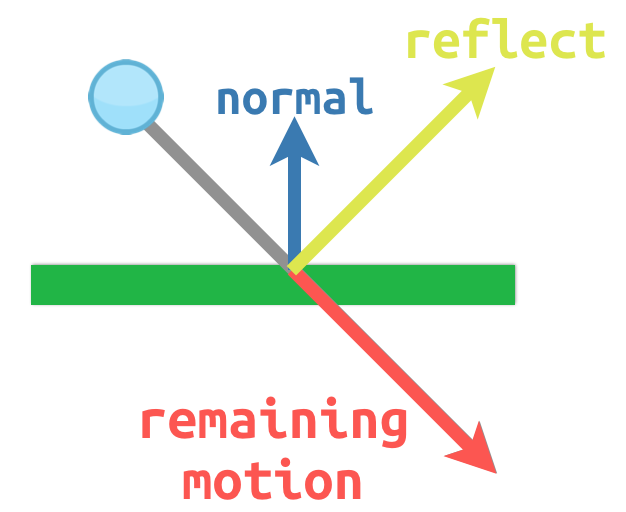The surface normal has a value of (0, -1) because this is a horizontal surface. When the ball collides, we take its remaining motion (the amount left over when it hits the surface) and reflect it using the normal. In Godot, the Vector2 class has a bounce() method to handle this. Here is a GDScript example of the diagram above using a KinematicBody2D:

# object "collision" contains information about the collision
var collision = move_and_collide(velocity * delta)
if collision:
var reflect = collision.remainder.bounce(collision.normal)
velocity = velocity.bounce(collision.normal)
move_and_collide(reflect)

// KinematicCollision2D contains information about the collision
KinematicCollision2D collision = MoveAndCollide(_velocity * delta);
if (collision != null)
{
var reflect = collision.Remainder.Bounce(collision.Normal);
_velocity = _velocity.Bounce(collision.Normal);
MoveAndCollide(reflect);
}


## Dot product¶

The dot product is one of the most important concepts in vector math, but is often misunderstood. Dot product is an operation on two vectors that returns a scalar. Unlike a vector, which contains both magnitude and direction, a scalar value has only magnitude.

The formula for dot product takes two common forms:eHowever, in most cases it is easiest to use the built-in method. Note that the order of the two vectors does not matter:

var c = a.dot(b)
var d = b.dot(a)  # these are equivalent

float c = a.Dot(b);
float d = b.Dot(a);  // these are equivalent


The dot product is most useful when used with unit vectors, making the first formula reduce to just cosθ. This means we can use the dot product to tell us something about the angle between two vectors: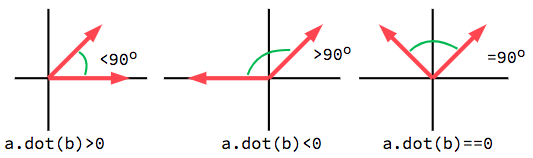When using unit vectors, the result will always be between -1 (180°) and 1 (0°).

### Facing¶

We can use this fact to detect whether an object is facing toward another object. In the diagram below, the player P is trying to avoid the zombies A and B. Assuming a zombie’s field of view is 180°, can they see the player?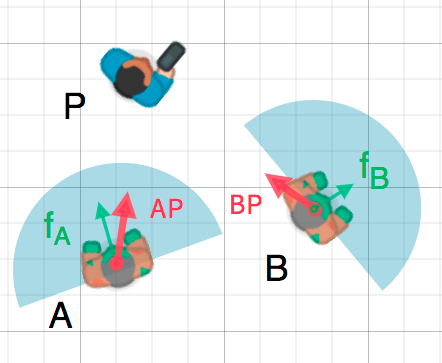The green arrows fA and fB are unit vectors representing the zombies’ facing directions and the blue semicircle represents its field of view. For zombie A, we find the direction vector AP pointing to the player using P - A and normalize it. If the angle between this vector and the facing vector is less than 90°, then the zombie can see the player.

In code it would look like this:

var AP = (P - A).normalized()
if AP.dot(fA) > 0:
print("A sees P!")

var AP = (P - A).Normalized();
if (AP.Dot(fA) > 0)
{
GD.Print("A sees P!");
}


## Cross product¶

Like the dot product, the cross product is an operation on two vectors. However, the result of the cross product is a vector with a direction that is perpendicular to both. Its magnitude depends on their relative angle. If two vectors are parallel, the result of their cross product will be a null vector.The cross product is calculated like this:

var c = Vector3()
c.x = (a.y * b.z) - (a.z * b.y)
c.y = (a.z * b.x) - (a.x * b.z)
c.z = (a.x * b.y) - (a.y * b.x)

var c = new Vector3();
c.x = (a.y * b.z) - (a.z * b.y);
c.y = (a.z * b.x) - (a.x * b.z);
c.z = (a.x * b.y) - (a.y * b.x);


With Godot, you can use the built-in method:

var c = a.cross(b)

var c = a.Cross(b);


Nota

In the cross product, order matters. a.cross(b) does not give the same result as b.cross(a). The resulting vectors point in opposite directions.

### Calculating normals¶

One common use of cross products is to find the surface normal of a plane or surface in 3D space. If we have the triangle ABC we can use vector subtraction to find two edges AB and AC. Using the cross product, AB x AC produces a vector perpendicular to both: the surface normal.

Here is a function to calculate a triangle’s normal:

func get_triangle_normal(a, b, c):
# find the surface normal given 3 vertices
var side1 = b - a
var side2 = c - a
var normal = side1.cross(side2)
return normal

Vector3 GetTriangleNormal(Vector3 a, Vector3 b, Vector3 c)
{
// find the surface normal given 3 vertices
var side1 = b - a;
var side2 = c - a;
var normal = side1.Cross(side2);
return normal;
}


### Pointing to a target¶

In the dot product section above, we saw how it could be used to find the angle between two vectors. However, in 3D, this is not enough information. We also need to know what axis to rotate around. We can find that by calculating the cross product of the current facing direction and the target direction. The resulting perpendicular vector is the axis of rotation.